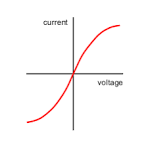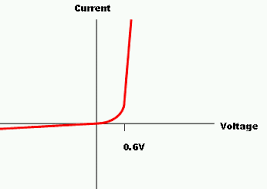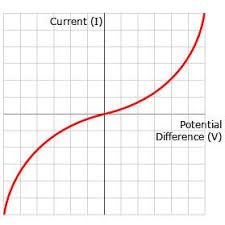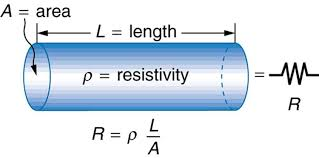# Physics Edexcel A Level 3: Electricity

?

## Ohm's Law

Ohm's Law: At a constant temperature, the current through a conductor is proportional to the p.d. applied across it

Non-Ohmic Components

• Filament Lamp• As the temperature increases, the resistance increases, as metals have a high resistance when hot. Higher resistance causes a lower gradient
1 of 9

## Ohm's Law 2/Diode

• Has a low resistance when 'forward biased', i.e. when v > 0.6. But when v < 0.6, the resistance becomes so high it is virtually non-conducting.
• 0.6V = threshold voltage
2 of 9

## Ohm's Law 3Thermistor

• Shown is a NTC thermistor, with the resistance decreasing at it gets hotter.
• This is due to a semi-conducting material, whose atoms release more conduction electrons as the temperature rises
3 of 9

## Conservation Laws

Quantity Series Rules Parallel Rules Current (I) Current is the same throughout (I1 = I2 = I3) Itotal = I1 +I2 + I3 Voltage (V) Vtotal = V1 +V2 + V3 Voltage is the same throughout (V1 = V2 = V3) Resistance (R) Rtotal = R1 +R2 + R3 1/Rtotal = 1/R1 +1/R2 + 1/R3

• Sum of the currents entering a junction = 0
• In a closed loop the emf sum = sum of p.ds (IR)
4 of 9

## Resistivity

• It is a property of materials that doesn't vary with the size, unlike resistance
• R = pl/A
• Experiment: Measuring wires of different lengths to plot a graph to find resistivity
• Using a screw-gauge to measure cross-sectional area
• Graph with length on x-axis, and resistance on y-axis, gradient p/A
• You could also vary the area instead, with the current on the x-axis, and gradient pl5 of 9

## Curr

I = nqva

• We use the drift velocity (v) as charged particles do not travel in a straight line through a conductor, because they collide with other particles in the material
• n = carrier density, how many charge carriers in the material

Carrier densities

• In metals, it is very large, which makes them good conductors as they have little resistivity
• Insulators have almost none, meaning they have very high resistivity
• Semiconductors have low amounts, meaning they have higher resistivity than metals
• LDRs are semiconductors, therefore is there is more light energy, more charge carriers are freed.
• As the temperature in an NTC thermistors, the charge carriers also increases. The lattice vibrations here have a very minimal effect.
• If the temperature is increased in a metal, there is an increase in lattice vibration, which reduces the drift velocity. Therefore the resistivity increases with temperature.
6 of 9

## E.M.F and Internal Resistance

• E = V+ Ir
• E = emf (total voltage in a cell)
• V = voltage of the circuit
• I = current
• r = internal resistance
• When a cell is connected and current is drawn, some of the energy is dissipated within the cell itself.
7 of 9

## Potential Divider Circuits

• Potential divider circuit:
• I = Vin / (R1 + R2)
• I = Vout / R1
• Vout = Vin x R/ (R1 + R2)
8 of 9

## Semiconductors

• Semiconductors start with a low amount of charge carriers, which are free electrons
• As the energy increases, the amount of charge carriers increases, due to the electrons passing amount of energy needed to break from the other atoms.
• This means that the current increases, therefore the resistivity decreases
9 of 9